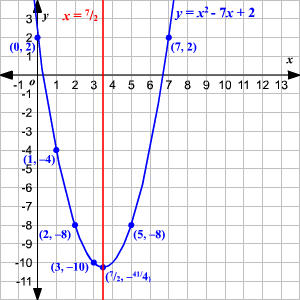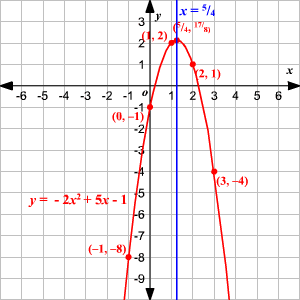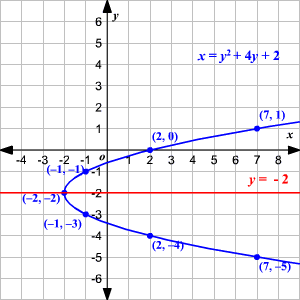# Graphing Quadratic Equations Using the Axis of Symmetry

A quadratic equation is a polynomial equation of degree $2$ .  The standard form of a quadratic equation is

$0=a{x}^{2}+bx+c$

where   $a,b$ and $c$ are all real numbers and $a\ne 0$ .

If we replace $0$ with $y$ , then we get a quadratic function

$y=a{x}^{2}+bx+c$

whose graph will be a parabola .

The axis of symmetry of this parabola will be the line $x=-\frac{b}{2a}$ . The axis of symmetry passes through the vertex, and therefore the $x$ -coordinate of the vertex is $-\frac{b}{2a}$ . Substitute $x=-\frac{b}{2a}$ in the equation to find the $y$ -coordinate of the vertex. Substitute few more $x$ -values in the equation to get the corresponding $y$ -values and plot the points. Join them and extend the parabola.

Example 1:

Graph the parabola $y={x}^{2}-7x+2$ .

Compare the equation with $y=a{x}^{2}+bx+c$ to find the values of $a$ , $b$ , and $c$ .

Here, $a=1,b=-7$ and $c=2$ .

Use the values of the coefficients to write the equation of axis of symmetry .

The graph of a quadratic equation in the form   $y=a{x}^{2}+bx+c$ has as its axis of symmetry the line $x=-\frac{b}{2a}$ . So, the equation of the axis of symmetry of the given parabola is $x=\frac{-\left(-7\right)}{2\left(1\right)}$ or $x=\frac{7}{2}$ .

Substitute $x=\frac{7}{2}$ in the equation to find the $y$ -coordinate of the vertex.

$\begin{array}{l}y={\left(\frac{7}{2}\right)}^{2}-7\left(\frac{7}{2}\right)+2\\ \text{\hspace{0.17em}}\text{\hspace{0.17em}}\text{\hspace{0.17em}}\text{\hspace{0.17em}}=\frac{49}{4}-\frac{49}{2}+2\\ \text{\hspace{0.17em}}\text{\hspace{0.17em}}\text{\hspace{0.17em}}\text{\hspace{0.17em}}=\frac{49\text{\hspace{0.17em}}-\text{\hspace{0.17em}}98\text{\hspace{0.17em}}+\text{\hspace{0.17em}}8}{4}\\ \text{\hspace{0.17em}}\text{\hspace{0.17em}}\text{\hspace{0.17em}}\text{\hspace{0.17em}}\text{\hspace{0.17em}}=-\frac{41}{4}\end{array}$

Therefore, the coordinates of the vertex are $\left(\frac{7}{2},-\frac{41}{4}\right)$ .

Now, substitute a few more $x$ -values in the equation to get the corresponding $y$ -values.

 $x$ $y={x}^{2}-7x+2$ $0$ $2$ $1$ $-4$ $2$ $-8$ $3$ $-10$ $5$ $-8$ $7$ $2$

Plot the points and join them to get the parabola.Example 2:

Graph the parabola $y=-2{x}^{2}+5x-1$ .

Compare the equation with $y=a{x}^{2}+bx+c$ to find the values of $a$ , $b$ , and $c$ .

Here, $a=-2,b=5$ and $c=-1$ .

Use the values of the coefficients to write the equation of axis of symmetry.

The graph of a quadratic equation in the form   $y=a{x}^{2}+bx+c$ has as its axis of symmetry the line $x=-\frac{b}{2a}$ . So, the equation of the axis of symmetry of the given parabola is $x=\frac{-\left(5\right)}{2\left(-2\right)}$ or $x=\frac{5}{4}$ .

Substitute $x=\frac{5}{4}$ in the equation to find the $y$ -coordinate of the vertex.

$\begin{array}{l}y=-2{\left(\frac{5}{4}\right)}^{2}+5\left(\frac{5}{4}\right)-1\\ \text{\hspace{0.17em}}\text{\hspace{0.17em}}\text{\hspace{0.17em}}\text{\hspace{0.17em}}=\frac{-50}{16}+\frac{25}{4}-1\\ \text{\hspace{0.17em}}\text{\hspace{0.17em}}\text{\hspace{0.17em}}\text{\hspace{0.17em}}=\frac{-50\text{\hspace{0.17em}}+\text{\hspace{0.17em}}100\text{\hspace{0.17em}}-\text{\hspace{0.17em}}16}{16}\\ \text{\hspace{0.17em}}\text{\hspace{0.17em}}\text{\hspace{0.17em}}\text{\hspace{0.17em}}\text{\hspace{0.17em}}=\frac{34}{16}\\ \text{\hspace{0.17em}}\text{\hspace{0.17em}}\text{\hspace{0.17em}}\text{\hspace{0.17em}}\text{\hspace{0.17em}}=\frac{17}{8}\end{array}$

Therefore, the coordinates of the vertex are $\left(\frac{5}{4},\frac{17}{8}\right)$ .

Now, substitute a few more $x$ -values in the equation to get the corresponding $y$ -values.

 $x$ $y=-2{x}^{2}+5x-1$ $-1$ $-8$ $0$ $-1$ $1$ $2$ $2$ $1$ $3$ $-4$

Plot the points and join them to get the parabola.Example 3:

Graph the parabola $x={y}^{2}+4y+2$ .

Here, $x$ is a function of $y$ . The parabola opens "sideways" and the axis of symmetry of the parabola is horizontal. The standard form of equation of a horizontal parabola is $x=a{y}^{2}+by+c$ where $a$ , $b$ , and $c$ are all real numbers and   $a\ne 0$ and the equation of the axis of symmetry is $y=\frac{-b}{2a}$ .

Compare the equation with $x=a{y}^{2}+by+c$ to find the values of $a$ , $b$ , and $c$ .

Here, $a=1,b=4$ and $c=2$ .

Use the values of the coefficients to write the equation of axis of symmetry.

The graph of a quadratic equation in the form   $x=a{y}^{2}+by+c$ has as its axis of symmetry the line $y=-\frac{b}{2a}$ . So, the equation of the axis of symmetry of the given parabola is $y=\frac{-4}{2\left(1\right)}$ or $y=-2$ .

Substitute $y=-2$ in the equation to find the $x$ -coordinate of the vertex.

$\begin{array}{l}x={\left(-2\right)}^{2}+4\left(-2\right)+2\\ \text{\hspace{0.17em}}\text{\hspace{0.17em}}\text{\hspace{0.17em}}\text{\hspace{0.17em}}=4-8+2\\ \text{\hspace{0.17em}}\text{\hspace{0.17em}}\text{\hspace{0.17em}}\text{\hspace{0.17em}}=-2\end{array}$

Therefore, the coordinates of the vertex are $\left(-2,-2\right)$ .

Now, substitute a few more $y$ -values in the equation to get the corresponding $x$ -values.

 $y$ $x={y}^{2}+4y+2$ $-5$ $7$ $-4$ $2$ $-3$ $-1$ $-1$ $-1$ $0$ $2$ $1$ $7$

Plot the points and join them to get the parabola.